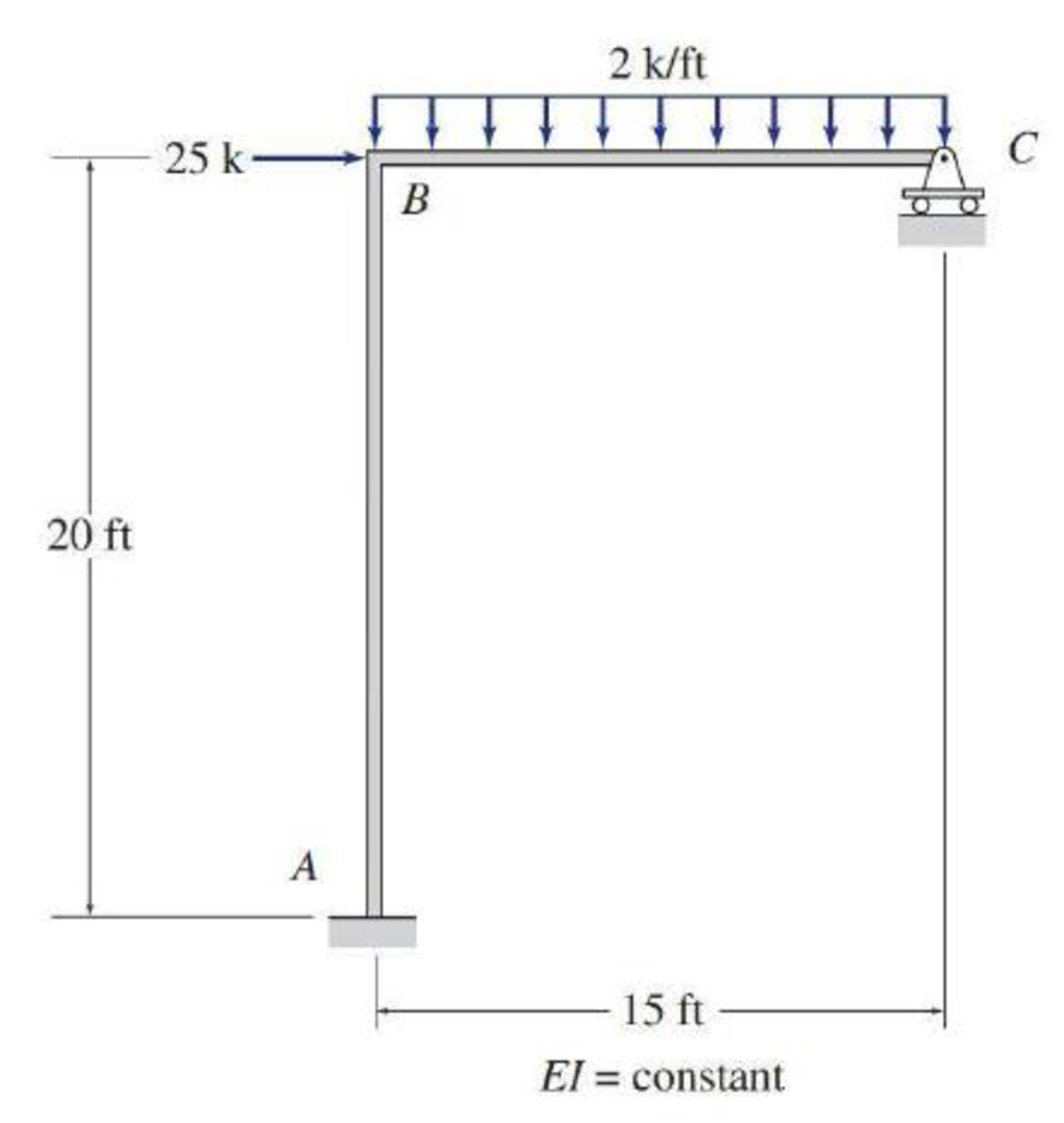# Determine the member end moments and reactions for the frames shown in Figs. P16.24–P16.31 by using the moment-distribution method. FIG. P16.24

#### Solutions

Chapter
Section
Chapter 16, Problem 24P
Textbook Problem
665 views

## Determine the member end moments and reactions for the frames shown in Figs. P16.24–P16.31 by using the moment-distribution method.FIG. P16.24

To determine

Find the member end moments and reactions for the frames.

### Explanation of Solution

Fixed end moment:

Formula to calculate the relative stiffness for fixed support IL and for roller support (34)(IL).

Formula to calculate the fixed moment for point load with equal length are PL8.

Formula to calculate the fixed moment for point load with unequal length are Pab2L2 and Pa2bL2.

Formula to calculate the fixed moment for UDL is WL212.

Formula to calculate the fixed moment for UVL are WL230 and WL220.

Formula to calculate the fixed moment for deflection is 6EIΔL2.

Calculation:

Consider the flexural rigidity EI of the frame is constant.

Show the free body diagram of the entire frame as in Figure 1.

Refer Figure 1,

Calculate the relative stiffness KBA for member AB of the frame as below:

KBA=I20

Calculate the relative stiffness KBC for member BC of the frame as below:

KBC=(34)I15=I20

Calculate the distribution factor DFBA for member BA of the frame.

DFBA=KBAKBA+KBC

Substitute I20 for KBA and I20 for KBC.

DFBA=I20I20+I20=0.5

Calculate the distribution factor DFBC for member BC of the frame.

DFBC=KBCKBA+KBC

Substitute I20 for KBA and I20 for KBC.

DFBC=I20I20+I20=0.5

Check for sum of distribution factor as below:

DFBA+DFBC=1

Substitute 0.5 for DFBA and 0.5 for DFBC.

0.5+0.5=1

Hence, OK.

Consider sideway prevented:

Calculate the fixed end moment for BC.

FEMBC=2×(15)212=37.5kft

Calculate the fixed end moment for CB.

FEMCB=2×(15)212=37.5kft

Show the calculation of M0 moments using moment distribution method for side-sway prevented as in Table 1.

Show the free body diagram of the member AB and BC with horizontal reaction for with unknown load as in Figure 2.

Refer Figure 2,

Consider member BC:

Calculate the vertical reaction at the joint B by taking moment about point A.

+MA=0Bx1(20)14.128.2=0Bx1(20)=42.3Bx1=42.320Bx1=2.12k

Calculate the horizontal reaction at joint A by resolving the horizontal equilibrium.

+Fx=0Ax1+Bx1=0Ax12.12=0Ax1=2.12k

R=Bx1+25=2.12+25=27.12k

Consider sideway permitted:

Show the translation of the frame as in Figure 3

Write the expression to calculate the Fixed-end moment at AB and BA.

FEMAB=FEMBA=6EIΔ'(20)2

Assume the Fixed-end moment at AB and BA as 100kft

### Still sussing out bartleby?

Check out a sample textbook solution.

See a sample solution

#### The Solution to Your Study Problems

Bartleby provides explanations to thousands of textbook problems written by our experts, many with advanced degrees!

Get Started

Find more solutions based on key concepts
VocabularyState the meaning of the following terms: One Clo _____ Metabolic Rate _____ Heating Value _____

Engineering Fundamentals: An Introduction to Engineering (MindTap Course List)

Determine the resultant of the position vectors A and B.

International Edition---engineering Mechanics: Statics, 4th Edition

What is the name of the wireless network to which you connected?

Enhanced Discovering Computers 2017 (Shelly Cashman Series) (MindTap Course List)

Create the relational diagram to show the relationship between EMPLOYEE and JOB.

Database Systems: Design, Implementation, & Management

What element is in stainless steel that makes it corrosion resistant?

Precision Machining Technology (MindTap Course List)

Why might organizations be willing to take on the risk of BYOD?

Network+ Guide to Networks (MindTap Course List)

If your motherboard supports ECC DDR3 memory, can you substitute non-ECC DDR3 memory?

A+ Guide to Hardware (Standalone Book) (MindTap Course List)

Describe the difference between using a leading and a trailing electrode angle.

Welding: Principles and Applications (MindTap Course List)